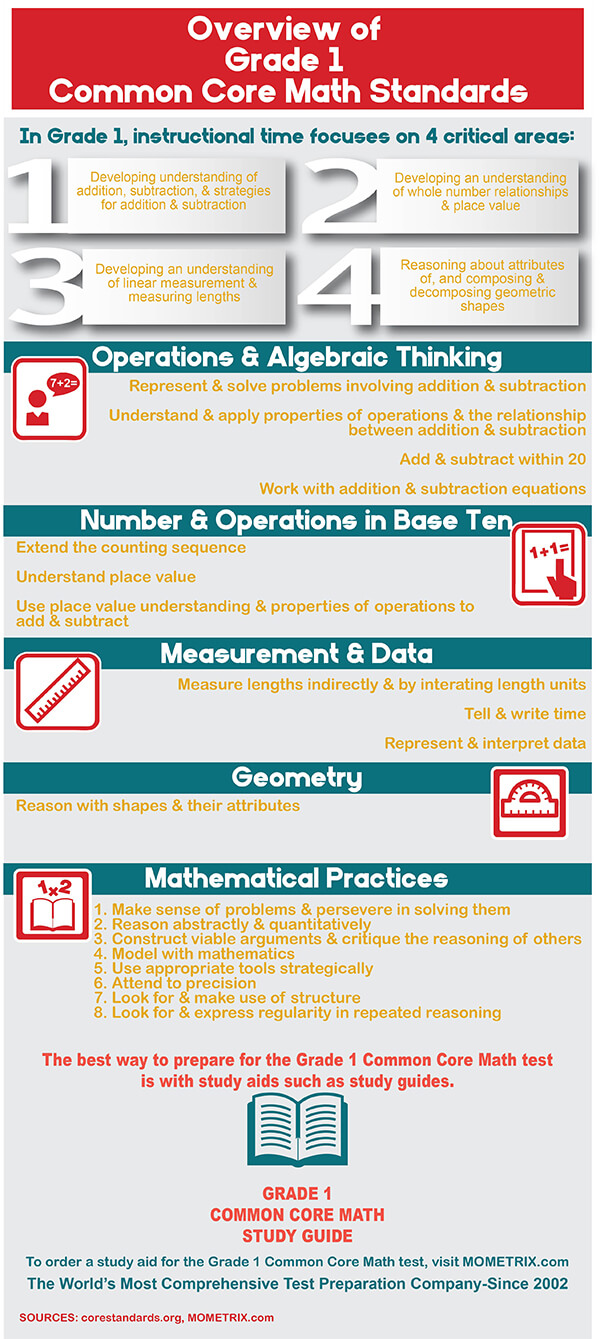# Mathematics Grade 1 Practice Questions

1. 2
2. 3
3. 5
4. 7
1. 3+12=?
2. 12-3=?
3. ?+3=12
4. 3+?=12
1. 2
2. 4
3. 6
4. 8
1. 9
2. 10
3. 11
4. 15
1. 2
2. 4
3. 16
4. 18
1. 7-5=2
2. 7+9=16
3. 9-7=2
4. 9+7=16
##### 7. Which of the following shows counting by 3’s?
1. 3, 3, 3, 3, 3, 3
2. 3, 5, 7, 9, 11
3. 3, 6, 9, 12, 15
4. 1, 2, 3, 1, 2, 3
##### 8. Which of the following shows 17-5 by counting?
1. 17, 16, 15, 14, 13, 12
2. 17, 15, 13, 11, 9, 7
3. 17, 18, 19, 20, 21, 22
4. 17, 19, 21, 23, 25, 27
1. 3
2. 6
3. 12
4. 18
##### 10. Subtract: 17-9=
1. 8
2. 9
3. 10
4. 11

1. B: The word “fewer” indicates the use of subtraction. 5-2=3.

2. A: All other choices represent the correct relationship between the number of t-shirts before and after her birthday.
B: 12-3=? The number of t-shirts she has now minus the number of t-shirts received at her birthday equals the number of t-shirts she had before her birthday.
C: ?+3=12 The number of t-shirts she had before her birthday plus the number of t-shirts received at her birthday equals the number of t-shirts she has now.
D: 3+?=12 The number of t-shirts received at her birthday plus the number of t-shirts she had before her birthday equals the number of t-shirts she has now.

3. C: Bobby had 4 books in his desk and he added 2 books. 4+2=6.

4. D: Add the number of games each friend has to find the total number of games. 5+4+6=15.

5. A: 18-8-8=10-8=2.

6. C: ?+7=9 and 9-7=? have the same meaning because both question marks are correctly replaced with 2.

7. C: Choice C shows 3, then 3+3=6, 6+3=9, 9+3=12, and 12+3=15.

8. A: Count down (subtract 1) five times, starting at 17: 17-1=16, 16-1=15, 15-1=14, 14-1=13, and 13-1=12.

9. D: Break 12 into 10+2 to add: 12+6=10+2+6=10+8=18.

10. A: Break 9 into parts of 7 and 2. Then subtract 7 and 2 from 17: : 17-9=17-7-2=10-2=8.